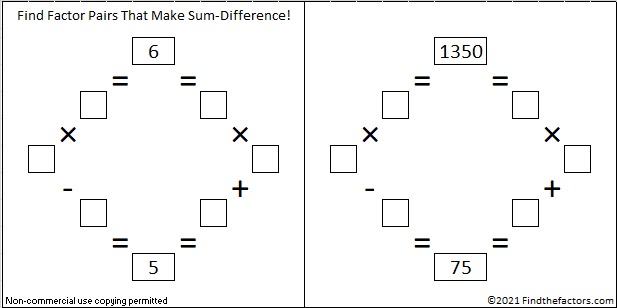# 1350 Logic is at the Heart of This Puzzle

Contents

### Today’s Puzzle:

By simply changing two clues of that recently published puzzle that I rejected, I was able to create a love-ly puzzle that can be solved entirely by logic. Can you figure out where to put the numbers from 1 to 12 in each of the four outlined areas that divide the puzzle into four equal sections? If you can, my heart might just skip a beat!

If you need some tips on how to get started on this puzzle, check out this video:

### Factors of 1350:

• 1350 is a composite number.
• Prime factorization: 1350 = 2 × 3 × 3 × 3 × 5 × 5, which can be written 1350 = 2 × 3³ × 5²
• The exponents in the prime factorization are 1, 3 and 2. Adding one to each and multiplying we get (1 + 1)(3 + 1)(2 + 1) = 2 × 4 × 3 = 24. Therefore 1350 has exactly 24 factors.
• Factors of 1350: 1, 2, 3, 5, 6, 9, 10, 15, 18, 25, 27, 30, 45, 50, 54, 75, 90, 135, 150, 225, 270, 450, 675, 1350
• Factor pairs: 1350 = 1 × 1350, 2 × 675, 3 × 450, 5 × 270, 6 × 225, 9 × 150, 10 × 135, 15 × 90, 18 × 75, 25 × 54, 27 × 50 or 30 × 45
• Taking the factor pair with the largest square number factor, we get √1350 = (√225)(√6) = 15√6 ≈ 36.74235

### Sum-Difference Puzzles:

6 has two factor pairs. One of those pairs adds up to 5, and the other one subtracts to 5. Put the factors in the appropriate boxes in the first puzzle.

1350 has twelve factor pairs. One of the factor pairs adds up to ­75, and a different one subtracts to 75. If you can identify those factor pairs, then you can solve the second puzzle!The second puzzle is really just the first puzzle in disguise. Why would I say that?

### More about the Number 1350:

1350 is the sum of consecutive prime numbers two ways:
It is the sum of the fourteen prime numbers from 67 to 131, and
673 + 677 = 1350

1350 is the hypotenuse of two Pythagorean triples:
810-1080-1350 which is (3-4-5) times 270
378-1296-1350 which is (7-24-25) times 54

1350 is also the 20th nonagonal number because 20(7 · 20 – 5)/2 = 1350

This site uses Akismet to reduce spam. Learn how your comment data is processed.